﻿ 现阶段我国外汇储备规模适度状况的多视角估测与比较

# 现阶段我国外汇储备规模适度状况的多视角估测与比较The Estimation & Comparison of Moderation of China’s Forex Reserve Scale at Present Phase from Various Perspectives

Abstract: By means of IMF’s quantitative index, comprehensive metrical standard, and classical Cost-benefit approach such as Argarwal model, this dissertation estimates the appropriateness of National Forex Reserve after neo-millennium, and the result indicates the conclusions of the three methods are in agreement, which epitomizes the economic commonness of Forex reserve. By making reference to foreign Monetary Authority experiences, the paper suggests several proposals for optimizing the scale of Chinese Forex reserve at last.

1. 引言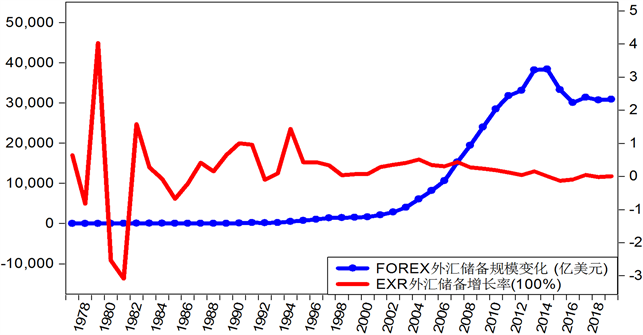Figure 1. Our national Forex reserve scale and its growth rate of the interim 1977-2019 per annum

2. 文献综述

3. 我国外汇储备规模的比例法分析

(一) IMF定量指标法

1) 近年来的实际储备趋势 = 实际外汇储备/国内生产总值，序列结果的均值 = 0.313473。然后按均值计算出2001~2019年各年的适度外汇储备规模，公式是：

2) 近年来的储备对进口额的比率=实际外汇储备/年进口额，序列结果的均值 = 1.595677。然后按均值计算出2001~2019年各年的适度外汇储备规模，公式是：

3) 近年来的储备对国际收支综合差额的比率 = 实际外汇储备/(短期外债余额 + 年进口额/4)，序列结果的均值 = 2.668531。然后按均值计算出2001~2019年各年的适度外汇储备规模，公式是：

Table 1. Conclusions of estimating the national Forex reserve moderation per IMF quantitative indexes for the interim of 2001-2019 (unit: hundreds millions dollar)

1、外汇储备/进口额比例分析Table 3. The ratio of Forex reserve to total import for the interim of 2001-2019 (unit: hundreds millions dollar)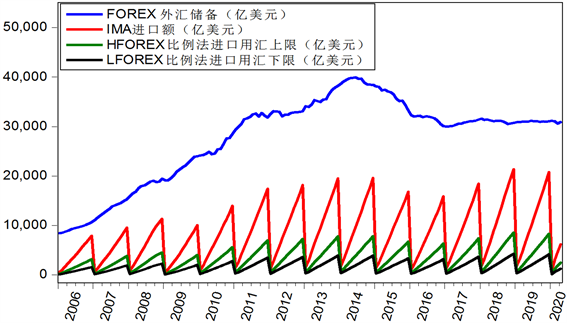Figure 2. The upper limit & lower limit of Forex for national import and forex reserve of the interim 2006-2020

2、外汇储备/短期外债比例分析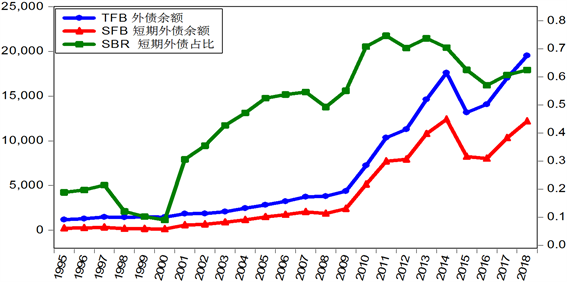Figure 3. The national short-term foreign debt ratio of the interim 1995-2018Table 4. The ratio of Forex to short-term foreign debt for the interim of 2001-2019

(二) IMF综合测量标准Table 5. Conclusions of estimating the national Forex reserve moderation per IMF comprehensive standard for the interim of 2001-2019 (unit: hundreds millions dollar)

4. 我国外汇储备规模的成本–收益法分析

$R=\frac{W}{\mathrm{ln}p}\left(\mathrm{ln}k+\mathrm{ln}{q}_{1}-\mathrm{ln}{q}_{2}\right)$

R：适度的外汇储备；

W：国际收支逆差额；

p：国际收支逆差出现的概率；

k：国内生产总值GDP∕全社会固定资产投资总额；

q1：追加资本的进口含量，可表示为：进口初级品(或者进口生产资料)∕新增社会固定资产投资；

q2：进口的生产性物品占总产出的比例，可以表示为：进口初级产品∕国内生产总值GDP。

(一) 扩展的阿格沃尔模型

$R=\frac{W}{\mathrm{ln}p}\left(\mathrm{ln}k+\mathrm{ln}{q}_{1}-\mathrm{ln}{q}_{2}\right)+\alpha \text{ }\text{ }\text{FD}+\beta \text{ }\text{ }\text{FDI}+\gamma \text{ }\text{ }\text{GDP}+\omega \text{ }\text{ }\text{ECA}+F$

K = GDP/全社会固定资产投资总额 = GDP/gi。

Gi = 全社会固定资产投资总额。

q1 = 进口初级品/新增固定资产投资 = mp/ngi。

mp = 初级产品进口额。

ngi = 新增固定资产投资。

q2 = 进口初级产品/GDP = mp/GDP。

FD：外债余额，是一个国家短期外债与长期外债之和。

FDI：境外投资者直接投资累计余额。

GDP：国内生产总值。

EXA：外汇市场交易额。

F：对抗风险及发展基金的用汇量。

(二) 扩展的阿格沃尔模型参数的确定

1、国际收支逆差额及其发生的概率

2、外债余额的还本付息率α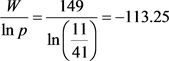Figure 4. Chinese foreign debt balance and liability ratio of the interim 1995-2018

Table 6. The national foreign debt balance and repayment ratio for the interim of 2007-2019

3、境外投资者撤资及利润汇出率βTable 7. The data of direct investment of financial institutions during the interim of 2012-2018 (unit: hundreds millions dollar)

4、个人用汇需求占GDP比率γTable 8. The Balance of Payments of China during the interim of 2015-2019 (unit: hundreds millions dollar)

5、央行干预外汇市场用汇量与外汇市场交易额的比率ω

2005年我国开始实行浮动汇率制，中央银行若要保持汇率波动不超过目标区间，就要提取一定量的外汇储备作为外汇平准基金，以备随时进行公开市场操作。参照此前实行固定汇率制时中央银行干预外汇市场的用汇量大约占中国外汇市场交易量的10%~20%的比例 ，我们保守地假定： $10%\le \omega \le 15%$

6、防范风险与应对危机的用汇量F

$\frac{W}{\mathrm{ln}p}=149/\mathrm{ln}\left(\frac{11}{41}\right)=-113.25$

$\begin{array}{c}{R}_{\mathrm{max}}=\frac{W}{\mathrm{ln}p}\left(\mathrm{ln}K+\mathrm{ln}{q}_{1}-\mathrm{ln}{q}_{2}\right)+\alpha \text{ }\text{ }\text{FD}+\beta \text{ }\text{ }\text{FDI}+\gamma \text{ }\text{ }\text{GDP}+\omega \text{ }\text{ }\text{EXA}+F\\ =-113.25×\left(\mathrm{ln}\left(\frac{\text{GDP}}{\text{GI}}\right)+\mathrm{ln}\left(\frac{\text{MP}}{\text{NGI}}\right)-\mathrm{ln}\left(\frac{\text{MP}}{\text{GDP}}\right)\right)\\ \text{\hspace{0.17em}}\text{\hspace{0.17em}}+0.13\text{\hspace{0.17em}}\text{FD}+0.12\text{\hspace{0.17em}}\text{FDI}+0.04\text{\hspace{0.17em}}\text{GDP}+0.15\text{\hspace{0.17em}}\text{EXA}+0.1Rh\end{array}$

${R}_{\mathrm{max}}=\frac{-113.25×\left(\mathrm{ln}\left(\frac{\text{GDP}}{\text{GI}}\right)+\mathrm{ln}\left(\frac{\text{MP}}{\text{NGI}}\right)-\mathrm{ln}\left(\frac{\text{MP}}{\text{GDP}}\right)\right)+0.13\text{\hspace{0.17em}}\text{FD}+0.12\text{\hspace{0.17em}}\text{FDI}+0.04\text{\hspace{0.17em}}\text{GDP}+0.15\text{\hspace{0.17em}}\text{EXA}}{0.9}$

${R}_{\mathrm{min}}=\frac{-113.25×\left(\mathrm{ln}\left(\frac{\text{GDP}}{\text{GI}}\right)+\mathrm{ln}\left(\frac{\text{MP}}{\text{NGI}}\right)-\mathrm{ln}\left(\frac{\text{MP}}{\text{GDP}}\right)\right)+0.11\text{\hspace{0.17em}}\text{FD}+0.09\text{\hspace{0.17em}}\text{FDI}+0.02\text{\hspace{0.17em}}\text{GDP}+0.1\text{\hspace{0.17em}}\text{EXA}}{0.95}$

${R}_{o}=\left({R}_{\mathrm{max}}+{R}_{\mathrm{min}}\right)/2$Table 9. The data for computing moderate Forex reserve scale per Agarwal model for the interim of 2001-2019 (unit: hundreds millions dollar)

2019年新增固定资产投资为负数，为适应外汇储备适度规模的对数计算公式，取绝对值。Table 10. The theoretical thresholds, moderate scale and actual reserve level of Chinese Forex reserve for the interim of 2001-2019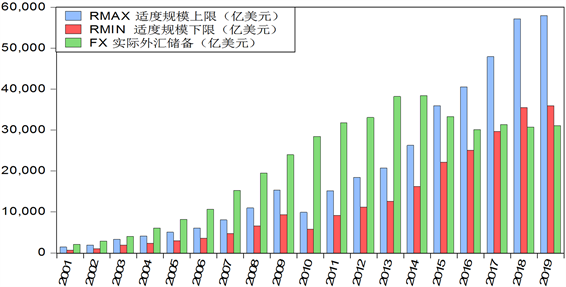Figure 5. The comparison between Forex moderate scale per Agarwal and its actual scale during the interim 2001-2019

5. 我国外汇储备规模适度状况的判定与改善措施Table 11. Comparison of the results IMF Quantitative index, Comprehensive meterage standard, and expanded Agarwal model

 韩继云. 我国外汇储备规模选择问题新探[J]. 南方经济, 1996(10): 26-28.

 Tariffin, R. (1961) Gold and the Dollar Crisis, Revised Edition. Yale University Press, New Haven.

 Aizenman & Marion (2004) Interactional Re-serve Holdings with Sovereignty Risk and Costly Tax Collection. Economic Journal, 114, 569-591.
https://doi.org/10.2139/ssrn.1288138

 王国林. 我国外汇储备适度状况分析[J]. 中国外汇管理, 2001(7): 15-17.

 王国林. 我国外汇储备适度状况定量分析[J]. 亚太经济, 2001(5): 49-52.

 曹文炼, 盛思鑫, 杨悦珉. 关于我国外汇储备下降过快的原因及合理规模的研究[J]. 全球化, 2017(4): 33-47.

 Heller, H.R. (1966) Optimal International Reserves. The Economic Journal, 76, 296-311.
https://doi.org/10.2307/2229716

 Agarwal, J.P. (1971) Optimal Monetary Reserves for Developing Countries. Review of World Economics, 1, 76-91.
https://doi.org/10.1007/BF02706047

 高丰, 于永达. 中国外汇储备对经济的影响及适度规模分析[J]. 金融与经济, 2003(6): 11-15.

 李静怡. 简析我国1994-2015年外汇储备适度规模[J]. 发展研究, 2017(5): 52-57.

 王凯, 庞震. 我国外汇储备减少的成因及有效管理策略研究[J]. 经济纵横, 2017(6): 75-81.

 罗素梅, 张逸佳. 中国的高额外汇储备可持续吗？[J]. 国际金融研究, 2015(4): 22-32.

 刘斌. 人民币自由兑换的外汇储备需求[J]. 财经研究, 2000(11): 59-64.

 凌超凡, 徐嘉欣. 正确认识我国外汇储备量的变动及管理策略[J]. 浙江金融, 2017(3): 19-24.

 International Monetary Fund (2011) Assessing Reserve Adequacy. Interna-tional Monetary Fund, Washington DC, 25. http://www.imf.org/external/np/sec/pn/2011/pn1147.htm
https://doi.org/10.5089/9781498339360.007

 Machlup, F. (1966) The Need for Monetary Reserves. Banca Nazionale Del Lavoro Quarterly Review, 19, 175-222.

 牛瑞芳. 后金融危机时代中国外汇储备规模和结构管理研究[J]. 商业时代, 2011(33): 52-53.

Top